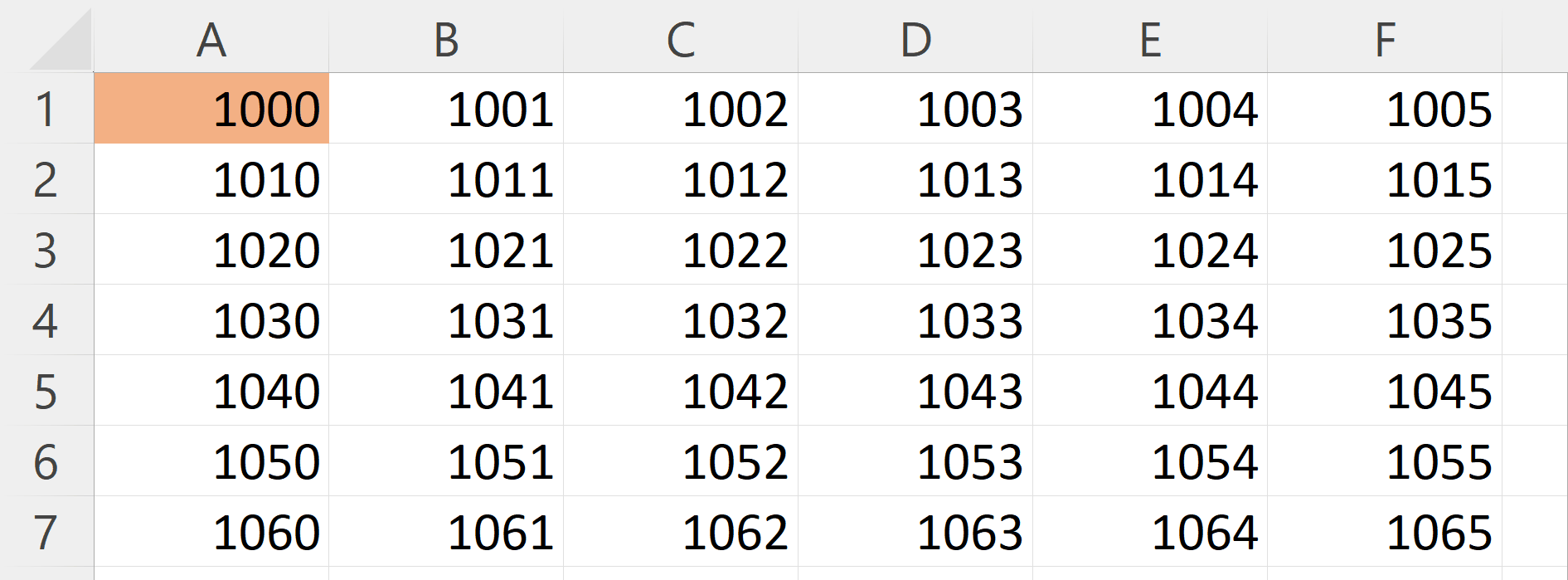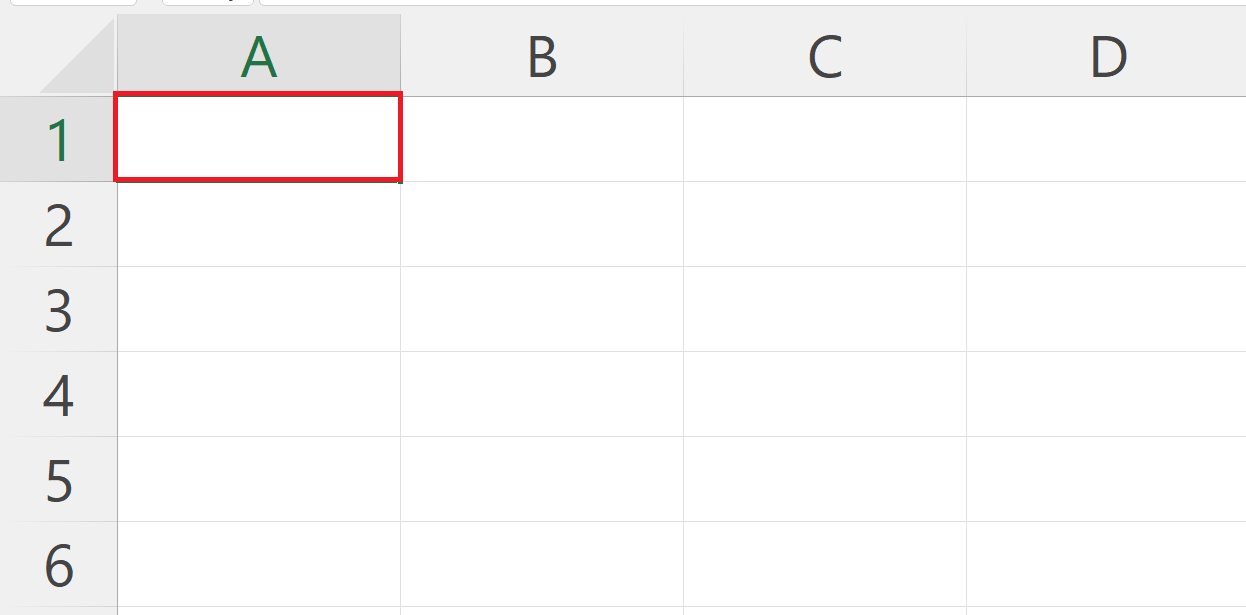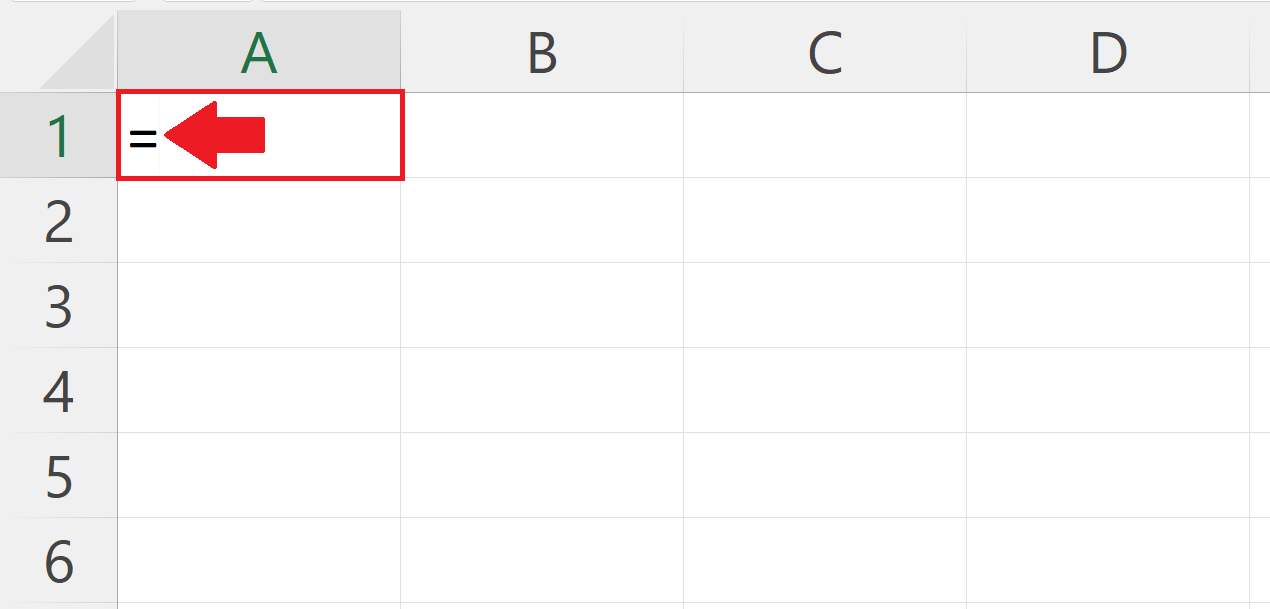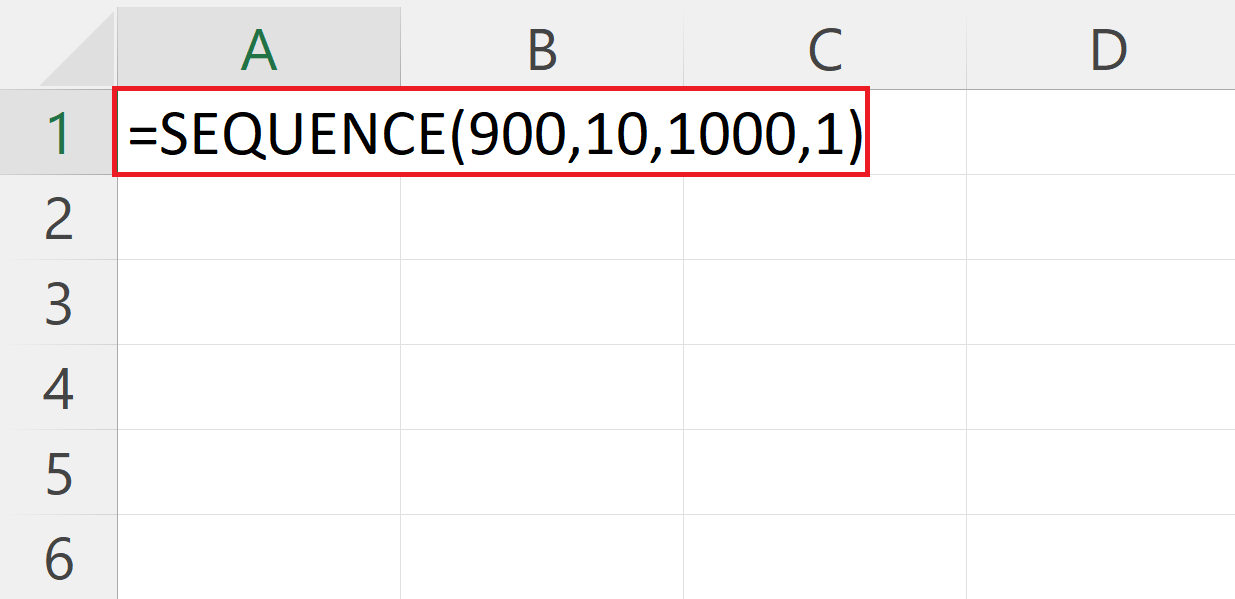# How to generate a list of all four digit number combinations in Microsoft Excel

In this we will learn how to generate all four digit numbers in Microsoft Excel. To generate all four digit number combinations there are multiple methods in Microsoft Excel. We will use the SEQUENCE function to generate all the four digit numbers. The SEQUENCE function is a dynamic array function that allows you to generate a sequence of values in a column or row. Its syntax is:

SEQUENCE(rows,columns,start,step)Four-digit combinations are important in Excel as they are commonly used in various applications such as generating passwords, creating unique identifiers, and tracking inventory or products. The four digit combinations can also be useful in statistical analysis, where a random sample of four-digit numbers can be selected to represent a larger population.

### Step 1 – Select a Blank Cell– Select a cell from where you want the list of all four digit number combinations to start.

### Step 2  – Place an Equals Sign### Step 3 – Use the SEQUENCE Function– The syntax of the SEQUENCE function will be :
SEQUENCE(900,10,1000,1)
– Where the first argument i.e. 900 is the number of rows in which the sequence is to be generated.
– The second argument i.e. 10 is the number of columns in which the sequence is to be generated.
– The third argument i.e. 1000 is the initial number from which  the sequence will start to be generated.
– The fourth argument i.e. 1 the step size in the sequence.

### Step 4 – Press the Enter Key– Press the Enter Key to generate the sequence.

### Step 5 – Check IF all the Combinations are Generated– Press the CTRL + END keys to jump to the last value of the generated sequence.